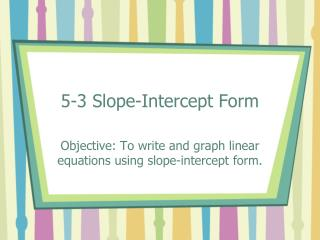DownloadDownload Presentation5-3 Slope-Intercept Form

# 5-3 Slope-Intercept Form

Download Presentation## 5-3 Slope-Intercept Form

- - - - - - - - - - - - - - - - - - - - - - - - - - - E N D - - - - - - - - - - - - - - - - - - - - - - - - - - -
##### Presentation Transcript

1. 5-3 Slope-Intercept Form Objective: To write and graph linear equations using slope-intercept form.

3. 5-3 Slope-Intercept Form • A linear equation is an equation that models a line. The variable is raised to the one power ONLY. • y = 2x is a line. • y = x2 is NOT A line (it’s a parabola) • y = 2x is NOT a line (it’s an exponential curve)

4. 5-3 Slope-Intercept Form • Graphs of linear functions may cross the y-axis at any point. A y-intercept is the y-coordinate of a point where the graph crosses the y-axis. What is the x-coordinate ALWAYS?

5. 5-3 Slope-Intercept Form Identify y-intercept and slope of each line:

6. 5-3 Slope-Intercept Form • The slope-intercept form of a linear equation of a non-vertical line is • y = mx + b • slope y-intercept

7. 5-3 Slope-Intercept Form • Example 1: What are slope and y-intercept: • Y = 5x -2 • y = mx + b • slope y-intercept • y = 5x - 2

8. 5-3 Slope-Intercept Form • Example 2: What is equation of the line with slope of and y - intercept of 7? • y = mx + b • slope y-intercept • y =

9. 5-3 Slope-Intercept Form • Example 3: Page 309 Which equation represents graph. • Find slope from two points. And y –intercept.

10. 5-3 Slope-Intercept Form • Example 4: What equation represents the line that passes through the points (2,1) and (5, -8) • Find slope from two points. • Use the what you know: slope and a point to find b. • Substitute m and b into equation for final answer.

11. 5-3 Slope-Intercept Form • Example 5: What is graph of y = 2x - 1 • Identify slope and y-intercept. • Graph y-intercept • Count off m () from b to get another point. Draw line.

12. 5-3 Slope-Intercept Form • HW; p. 312 9 – 42 every third